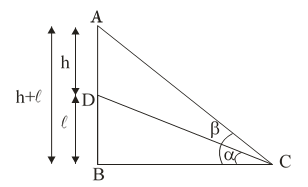#### A vertical tower stands on a horizontal plane and is surmounted by a vertical flagstaff of height h. At a point on the plane, the angles of elevation of the bottom and the top of the flagstaff are α and β, respectively. Prove that the height of the tower isAccording to questionHere h is the height of flagstaff AD.
Let  is the height of the tower
and  be the angle of elevation of the bottom and the top of the flagstaff.
In BDC

In ABC

equate equation (1) and (2) we get

[by cross multiplication]

Hence Proved